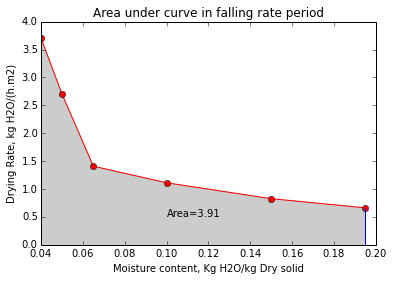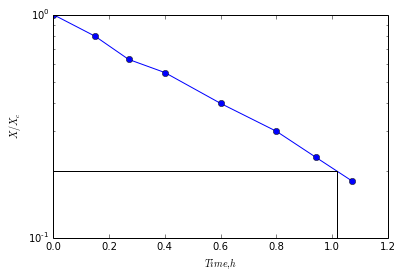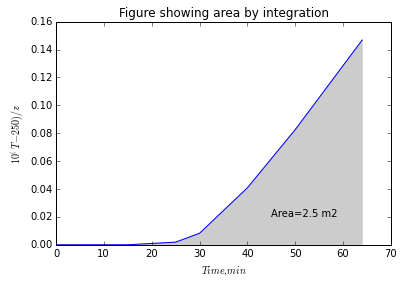# Chapter 9: Drying of Process Material¶

## Example 9.3-1 Page Number 526¶

In :
#Humidity from Vapor-pressure Data

#Variable declaration
Pas = 3.5                   #Vapor pressure of water at 26.7 deg C (kPa)
Pa = 2.76                   #Partial pressure of water vapor (kPa)
P = 101.3                   #Pressure at room temperature (kPa)

#Calculation
#Calculation for part (a)
H = 18.02*Pa/(28.97*(P - Pa))
#Calculation for part (b)
Hs = 18.02*Pas/(28.97*(P - Pas))
Hp = 100.*H/Hs
#Calculation for part (c)
Hr = 100.*Pa/Pas
#Result
print "(a) The Humidity is",round(H,5),"kg H2O/(kg dry air)"
print "(b) The saturation humidity is",round(Hs,5),"kg H2O/(kg dry air)"
print "(b) The percentage humidity is",round(Hp,1),"%"
print "(c) The precentage relative humidity is",round(Hr,1),"%"

(a) The Humidity is 0.01742 kg H2O/(kg dry air)
(b) The saturation humidity is 0.02226 kg H2O/(kg dry air)
(b) The percentage humidity is 78.3 %
(c) The precentage relative humidity is 78.9 %


## Example 9.3-2 Page Number 528¶

In :
#Use of Humidity Chart

#Variable declaration
T = 60.                        #Dry bulb temperature of air (deg C)
T_F = 140.                     #Temperature in FPS units (deg F)
Td = 26.7                      #Dew point of air (deg C)

#Calculation
H = 0.0225                     #Actual humid heat (Value determined from the Psychrometric chart)

Cs_SI = 1.005 + 1.88*H
Cs_Eng = 0.24 + 0.45*H
Vh_SI = (2.83e-3 + 4.56e-3*H)*(T + 273.)
Vh_Eng = (0.0252 + 0.0405*H)*(T_F + 460.)

#Result
print 'Actual humidity determine using Humidity chart= %5.4f kgH2O/kgdry air'%H
print "The calculated Humid Heat in SI units is",round(Cs_SI,3),"kJ/(kg dry air.K)"
print "The calculated Humid Heat in English units is",round(Cs_Eng,3),"btu/(lbm dry air.°C)"
print "The calculated Humid Volume in SI units is ",round(Vh_SI,3),"m3/kg dry air"
print "The calculated Humid Volume in English units is",round(Vh_Eng,2),"ft3/lbm dry air"

Actual humidity determine using Humidity chart= 0.0225 kgH2O/kgdry air
The calculated Humid Heat in SI units is 1.047 kJ/(kg dry air.K)
The calculated Humid Heat in English units is 0.25 btu/(lbm dry air.°C)
The calculated Humid Volume in SI units is  0.977 m3/kg dry air
The calculated Humid Volume in English units is 15.67 ft3/lbm dry air


## Example 9.3-3 Page Number 531¶

In :
#Adiabatic Saturation of Air

#Variable declaration
Ha = 0.030                        #Humidity of dry air (kg H2O/kg dry air)

#Calculation
#Calculation for (a)
Hb = 0.0500       #Value determined from Humidity chart for 90 % saturation (kg H2O/kg dry air)
T = 42.5          #Value determined from Humidity chart for 90 % saturation (deg C)
#Calculation for (b)
Hb = 0.0505       #Value determined from Humidity chart for 100 % saturation (kg H2O/kg dry air)
Tb = 40.5         #Value determined from Humidity chart for 90 % saturation (deg C)
#Result
print "(a) The value of humidity at 90 % saturation is ",Ha,"(kg H2O/kg dry air)"
print "    The value of temperature at 90 % saturation is ",T,"deg C"
print "(b) The value of humidity at 100 % saturation is ",Hb,"(kg H2O/kg dry air)"
print "    The value of temperature at 100 % saturation is ",Tb,"deg C"

(a) The value of humidity at 90 % saturation is  0.03 (kg H2O/kg dry air)
The value of temperature at 90 % saturation is  42.5 deg C
(b) The value of humidity at 100 % saturation is  0.0505 (kg H2O/kg dry air)
The value of temperature at 100 % saturation is  40.5 deg C


## Example 9.3-4 Page Number 532¶

In :
#Wet Bulb Temperature and Humidity

#Variable declaration
T = 60.               #Dry bulb temperature of vapor-air mixture (deg C)
Tw = 29.5             #Obtained wet bulb temperature (deg C)

#Calculation
H = 0.0135   #Humidity obtained from the adiabatic saturation curve (kg H2O/kg dry air)

#Result
print "The humidity obtained from the adiabatic curve is",H,"(kg H2O/kg dry air)"

The humidity obtained from the adiabatic curve is 0.0135 (kg H2O/kg dry air)


## Example 9.6-1 Page Number 541¶

In :
#Time of Drying from Drying Curve

#Variable declaration
X1 = 0.38                      #Initial Moisture content (kg H2O/kg dry solid)
X2 = 0.25                      #Final Moisture content (kg H2O/kg dry solid)
t1 = 1.28                      #Time required for drying solid (h) (Value determined from fig. 9.5-1A)
t2 = 3.08                      #Time required for drying solid (h) (Value determined from fig. 9.5-1A)

#Calculation
t = t2 - t1
#Result
print "The time required is ",t,"h"

The time required is  1.8 h


## Example 9.6-2 Page Number 541¶

In :
#Time of Drying from Drying Curve

#Variable declaration
X1 = 0.38                      #Initial Moisture content (kg H2O/kg dry solid)
X2 = 0.25                      #Final Moisture content (kg H2O/kg dry solid)
LsbyA = 21.5                   #From Fig.9.5-1b
Rc = 1.51                      #From Fig.9.5-1b
#Calculation
t = LsbyA*(X1 - X2)/Rc
#Result
print "The time required is ",round(t,2),"h"

The time required is  1.85 h


## Example 9.6-3 Page Number 543¶

In :
#Prediction of Constant-Rate Drying

#Varialbe declaration
A_eng = 1.5*1.5                   #Area of pan (ft2)
H = 0.01                          #Humidity of air stream (kg H2O/kg dry air) or (lbm H2O/lbm dry air)
Hw = 0.026                        #Saturated Humidity (kg H2O/kg dry air) or (lbm H2O/lbm dry air)

#Variable declaration SI units
A = 0.457*0.457                   #Area of pan (m2)
T = 65.6                          #Dry bulb temperature (deg C)
Tw = 28.9                         #Wet bulb temperature (deg C)
v = 6.1                           #Velocity of air stream flowing parallel to the surface (m/s)
lambdaw = 2433.                   #Value determined from steam table (kJ/kg)
lambdaw_eng = 1046.               #Value determined from steam table (btu/lbm)

#Calculation
Vh = (2.83e-3 + 4.56e-3*H)*(T + 273.)
Rho = (1. + H)/0.974
G = v*3600.*Rho
h = 0.0204*G**0.8
Tw = 28.9
Rc_SI = (h/(lambdaw*1000.))*(T - Tw)*3600.
R = Rc_SI*A

print "Results in SI units"
print "The calculated total rate of evaporation in SI Units ",round(R,3),"kg H2O/h"

#Variable declaration English units
T_eng = 150.                      #Dry bulb temperature (F)
Tw = 28.9                         #Wet bulb temperature (deg C)
v_eng = 20.                       #Velocity of air stream flowing parallel to the surface (ft/s)
lambdaw_eng = 1046.               #Value determined from steam table (btu/lbm)
Tw_eng = 84.

#Calculation
Vh_eng = (0.0252 + 0.0405*H)
Rho_eng = 0.0647
G_eng = v_eng*3600.*Rho_eng
h_eng = 0.0128*G_eng**0.8
Rc_Eng = h_eng*(T_eng - Tw_eng)/lambdaw_eng
R_eng = Rc_Eng*A_eng

#Result
print "Results in English units"
print "The calculated total rate of evaporation in English Units",round(R_eng,3),"lbm H2O/h"

Results in SI units
The calculated total rate of evaporation in SI Units  0.708 kg H2O/h
Results in English units
The calculated total rate of evaporation in English Units 1.563 lbm H2O/h


## Example 9.7-1 Page Number 545¶

In :
#Graphical Integration in Falling-Rate Drying Period
import numpy as np
from scipy.interpolate import interp1d
import scipy.integrate as integrate
from matplotlib.pylab import plot,fill_between

#Variable declaration
X1 = 0.38             #Free moisture content (kg H2O/kg dry soild)
X2 = 0.04             #Dry solid (kg H2O/kg dry soild)
Ls = 399.             #Weight of dry solid (kg)
A = 18.58             #Area of top drying surface (m2)

Xc = 0.195            #Critical free moisture content (kg H2O/kg dry soild)
Rc = 1.51
#Calculation
LsbyA = Ls/A
tc = LsbyA*(X1 - Xc)/Rc                     #Time required for drying under constant rate period

X = np.array([0.195,0.150,0.1,0.065,0.05,0.04])
R = np.array([1.51,1.21,0.9,0.71,0.37,0.27])
InvR = 1/R
plot(X,InvR,'ro-')
f = interp1d(X,InvR)
AUC = -integrate.simps(InvR,X)        #Time required for drying under falling rate period
tf = AUC*LsbyA

tfs = str(round(tf,2))
plot([Xc,Xc],[0.,InvR])
plot([X2,X2],[0.,InvR[len(X)-1]])
xlabel("Moisture content, Kg H2O/kg Dry solid")
ylabel("Drying Rate, kg H2O/(h.m2)")
fill_between(X,InvR,0,color='0.8')
#Result
print "Total drying time:", round(tc+tf,3), "h"
title('Area under curve in falling rate period')
text(0.1,0.5,'Area='+tfs)

print 'Because of numerical integration answer is different than graphical'

Total drying time: 6.536 h

Out:
<matplotlib.text.Text at 0x63fdd70>## Example 9.7-2 Page Number 547¶

In :
#Approximation of Straight Line for Falling-Rate Period

#Variable declaration
Rc = 1.51
Xc = 0.195            #Critical free moisture content (kg H2O/kg dry soild)
X2 = 0.040            #Dry solid (kg H2O/kg dry soild)
Ls = 399.             #Weight of dry solid (kg)
A = 18.58             #Area of top drying surface (m2)
LsbyA = Ls/A

#Calculation
t = LsbyA*Xc*log(Xc/X2)/(Rc)
#Result
print "The falling rate period is",round(t,2),"h"

The falling rate period is 4.39 h


## Example 9.8-1 Page Number 550¶

In :
#Constant-Rate Drying When Radiation and Convention are Present
import numpy as np
from scipy.interpolate import interp1d
from scipy.optimize import root

#Variable declaration
t2 = np.array([293,298,303,308,313,318,323])
Hs = np.array([14.8,20.2,27.6,36.,49.,65.,87.])

t1 = np.array([280,285,290,295,300,305,310,315,320,325,330,335,340])
lambds = np.array([2485.4,2473.9,2462.2,2450.3,2438.4,2426.3,2414.3,2402.0,2389.8,2377.6,2365.3,2353.0,2340.5])

T = 65.6                #Temperature of the bottom metal surface (deg C)
Zs = 0.0254             #Depth of the pan (m)
Km = 43.3               #Thermal conductivity of pan (W/m.K)
Ks = 0.865              #Thermal conductivity of material (W/m.K)
Zm = 0.00061            #Thickness of the metal pan (m)
e = 0.92                #Emissivity of the solid
H = 0.010               #Humidity of dry air (kg H2O/kg dry air)
Tw = 28.9               #Wet bulb temperature (deg C)
Ts = 32.2
Tr = 93.3               #Temperature of the surface (deg C)
lams = 2424.            #Value determined from steam table (kJ/kg)
tsg = 32.2
u = 6.1                 #m/s

#Calculation
fHs = interp1d(t2,Hs)
flambd = interp1d(t1,lambds)
T1 = Tr + 273.2
T2 = Ts + 273.2
cs = (1.005 + 1.88*H)*1e3
Rho = (1. + H)/0.974
G = u*3600.*Rho
hc = 0.0204*G**0.8
UK = 1/(1/hc+Zm/Km+Zs/Ks)
er = 1.02
Tsg = 303.5
while er >= 0.1:
print "Watch function value displyed to fall near to zero"
Tsg = float(raw_input("Enter the guess temperature  ")) + 273.2
hr = e*5.676*((T1/100)**4-((Tsg)/100)**4)/(T1-(Tsg))
Hs = fHs(Tsg)*1e-3
lambdas = flambd(Tsg)*1e3
k1 = (Hs-0.01)*lambdas/cs
k2 = 1+UK/hc
k3 = hr/hc
f = lambda tsc: -k1 + k2*(T-tsc) + k3*(Tr-tsc)
sol = root(f,T)
tsc = sol.x
er = abs(Tsg-(tsc+273.2))
print er
if er <= 0.01: break
Tsg = tsc+273.2

Rc = ((hc+UK)*(T-tsc)+hr*(Tr-tsc))*3600./lambdas
#Result

print "Rate of constant drying to ", round(Rc,2),"kg/(h.m2)"

Watch function value displyed to fall near to zero
Enter the guess temperature  34.4
6.79133441651
Watch function value displyed to fall near to zero
Enter the guess temperature  32.5
0.0923167102895
Rate of constant drying to  4.86 kg/(h.m2)


## Example 9.9-1 Page Number 553¶

In :
#Drying slabs of wood when Diffusion of Moisture Controls

#Variable declaration
Dl = 2.97e-6         #Experimental average diffusion cofficient (m2/h)
X_ = 0.04             #Moisture content of wood (kg H2O/kg dry wood)
Xt1 = 0.29           #Initial average moisture content
Xt = 0.09            #Final average moisture content
d = 25.4             #Thickness of the wooden plank (mm)
#Calculation
X1 = Xt1 - X_         #The free moisture content
X = Xt - X_
x1 = d/(2*1000.)
t = 4*(x1**2)*log(8*X1/((pi**2)*X))/((pi**2)*Dl)
Ea = X/X1
Dltbyx12 = 0.56
t_g = x1**2*Dltbyx12/Dl
#Result
print "The time needed for drying by calculation is",round(t,1),"h"
print "The time needed for drying by graph is",round(t_g,1),"h"

The time needed for drying by calculation is 30.8 h
The time needed for drying by graph is 30.4 h


## Example 9.9-2 Page Number 555¶

In :
#Diffusion Coefficient in the Tapoica Root
import numpy as np
import matplotlib.pyplot as plt
from scipy.interpolate import interp1d
from scipy.optimize import root
#Variable declaration
XbyXc = np.array([1.,0.8,0.63,0.55,0.4,0.3,0.23,0.18])
t = np.array([0.,0.15,0.27,0.40,0.6,0.8,0.94,1.07])
xbyxc = 0.2

#Calculation
x1 = 3./2.
plt.gca().set_yscale('log')
f = interp1d(t,XbyXc)
f1 = lambda tt: f(tt)-xbyxc
sol = root(f1,.97)
txbyxc = sol.x

plot(t,XbyXc,'bo-')
plot([0.0,txbyxc,txbyxc],[0.2,0.2,0.1],'k')
#plot([txbyxc,txbyxc],[0.2,0.1],'k')
xlabel('$Time, h$')
ylabel('$X/X_c$')
# for xbyxc = 0.2 from fig 9.9-1 Dl*t/x**2 = 0.56
absc = 0.56
DL =absc*x1**2/(txbyxc*3600)
#Result
print "Time required for drying to", xbyxc,"is ", round(txbyxc,2),"hr"
print 'Average moisture diffusivity %6.2e' %(DL),'m2/s'

Time required for drying to 0.2 is  1.02 hr
Average moisture diffusivity 3.44e-04 m2/s## Example 9.10-1 Page Number 559¶

In :
#Through Circulation Drying in a Bed
import numpy as np
from scipy.interpolate import interp1d

#Variable declaration
Xt1 = 1.                  #Initial total moisture content (kg H2O/kg dry air)
Xs = 0.01                 #The equilibrium moisture content (kg H2O/kg dry air)
Rho = 1602.               #Density of dry solid (kg/m3)
Rhos = 641.               #Density of dry solid (kg/m3)
H1 = 0.04                 #Inlet air humidity (kg H2O/kg dry air)
T1 = 121.1                #Temperature of air (deg C)
v = 0.811                 #Gas superficial velocity (m/s)
Xtc = 0.5                 #The total critical moisture content
Xt = 0.1                  #Final total moisture content (kg H2O/kg dry air)
x1 = 0.0508               #Depth of packed cylinders
mu = 2.15e-5              #Viscosity of air, kg/(m.s)

T = np.array([280,285,290,295,300,305,310,315,320,325,330,335,340])
lambds = np.array([2485.4,2473.9,2462.2,2450.3,2438.4,2426.3,2414.3,2402.0,2389.8,2377.6,2365.3,2353.0,2340.5])

#Calculation

flambd = interp1d(T,lambds)
lambdaw = flambd(320.2)
X1 = Xt1 - Xs
Xc = Xtc - Xs
X = Xt - Xs
Vh = (2.83e-3 + 4.56e-3*H1)*(T1 + 273.)
RhoH = (1. + H1)/Vh
Ga = v*RhoH*3600.*(1./(1.+ H1))
H = 0.05                  #Approximate average
Gt = (2459. + 2459.*H)/3600.
e = 1 - Rhos/Rho          #Void fraction (m3)
h = 0.0254                #The length of solid cylinder (m)
Dc = 0.00635              #Diameter
a = 4*(1 - e)*(h + 0.5*Dc)/(Dc*h)
Dp = (Dc*h + 0.5*Dc**2)**0.5
Nre = Dp*Gt/mu
h = 0.151*(Gt*3600)**0.59/Dp**0.41
Tw = 47.2
Cs = (1.005 + 1.88*H)*1000.
G = Ga/3600.
tc = Rhos*lambdaw*1000.*x1*(X1 - Xc)/(G*Cs*(T1 - Tw)*(1 - exp(-h*a*x1/(G*Cs))))
tf = Rhos*lambdaw*1000.*x1*Xc*log(Xc/X)/(G*Cs*(T1 - Tw)*(1 - exp(-h*a*x1/(G*Cs))))
t = tc+tf

#Result
print "Total drying time", round(t/3600,3),"h or", round(t),"s"

Total drying time 0.628 h or 2260.0 s


## Example 9.10-2 Page Number 562¶

In :
#Heat Balance on a Dryer
from scipy.optimize import root

#Variable declaration
Ls = 453.6       #Feed rate,kg of dry solids/h
X1 = 0.04        #moisture content in feed,kg of moisture/kg dry solids
X2 = 0.002       #moisture content in feed,kg of moisture/kg dry solids
cpA = 4.187      #Specific Heat of moisture, kJ/(kg water.K)
cpS = 1.465      #Specific Heat of solid, kJ/(kg dry solid.K)
Ts1 = 26.7       #Temperature of feed at inlet, °C
Ts2 = 62.8       #Temperature of product at outlet, °C
Tg2 = 93.3       #Temperature of air at inlet, °C
Tg1 = 37.8       #Temperature of air at outlet, °C
H2 = 0.01        #Humidity of incomming air, kg water/kg dry air
Tref = 0.0       #Reference Temperature, °C

#Calculation
#Enthalpy of Entering Air,
#Latent heat of water from steam table = 2501 kJ/kg @93.3°C
lambda0 = 2501.
cs2 = 1.005 + 1.88*H2
Hg2 = cs2*(Tg2-Tref) + lambda0*H2
Hs1 = cpS*(Ts1-Tref)+X1*cpA*(Ts1-Tref)
Hs2 = cpS*(Ts2-Tref)+X2*cpA*(Ts2-Tref)
We = Ls*(X2-X1)               #Water Transfered to air
delHs = Ls*(Hs1-Hs2)          #Enthalpy change of solid
a = 37.99     #Constants in simplified relation for Humidity dependence of Enthalpy of leaving gas
b = 2572.
er = 5.
h = 0.011
while er>=0.001:
g = We/(H2-h)
hc = ((g*Hg2+delHs)/g-a)/b
gc = We/(H2-hc)
er = (abs(h-hc)+abs(g-gc))/2
h = hc
g = gc

#Results
print "Air flow rate",round(g,2), "kg Dry Air/h"
print "Humidity of air leaving",round(h,4), "kg of Water/kg Dry Air"

Air flow rate 1170.92 kg Dry Air/h
Humidity of air leaving 0.0247 kg of Water/kg Dry Air


## Example 9.12-1 Page Number 573¶

In :
#Sterlization of Cans

#Variable declaration
t1 = 20.
t2 = 40. - 20.
t3 = 73. - 40.
T1 = 160.
T2 = 210.
T3 = 230.
T1_C = 71.1
T2_C = 98.9
T3_C = 110.
z = 18.
z_SI = 10.
#Calculation
t0_eng = t1*10.**((T1 - 250.)/z) + t2*10.**((T2 - 250.)/z) + t3*10.**((T3 - 250.)/z)
t0_SI = t1*10.**((T1_C - 121.1)/z_SI) + t2*10.**((T2_C - 121.1)/z_SI) + t3*10.**((T3_C - 121.1)/z_SI)

#Result
print "The calculated time of sterlization in English units is ",round(t0_eng,2),"min"
print "The calculated time of sterlization in SI units is ",round(t0_SI,2),"min"

The calculated time of sterlization in English units is  2.68 min
The calculated time of sterlization in SI units is  2.68 min


## Example 9.12-2 Page Number 574¶

In :
#Thermal Process Evaluation by Graphical Integration
import numpy as np
from scipy.interpolate import interp1d
import matplotlib.pyplot as plt

#Variable declaration
t = np.array([0.,15.,25.,30.,40.,50.,64.])
T = np.array([80.,165.,201.,212.5,225.,230.5,235.])
F0 = 2.45
z = 18
#Calculation
y = 10**((T-250.)/z)
f = interp1d(t,y,'cubic')
plt.plot(t,y)
plt.fill_between(t,y,0,color='0.8')
xlabel('$Time, min$')
ylabel('$10^(T-250)/z$')
title('Figure showing area by integration')
txt = 'Area='+str(round(I,1))+' m2'
text(45,0.02,txt)
#Result

print "The process time required is ",round(I,1),"is greater than", F0,"hence sterilization is adequet"

The process time required is  2.5 is greater than 2.45 hence sterilization is adequet## Example 9.12-3 Page Number 576¶

In :
#Pasteurization of Milk
#Variable declaration
F150 = 9.              #Typical value (min)
D150 = 0.6
#Calculation
N0byN = 10.**(F150/D150)
#Result
print 'The reduction in number of viable cells is %10.3e'%(N0byN)

The reduction in number of viable cells is  1.000e+15